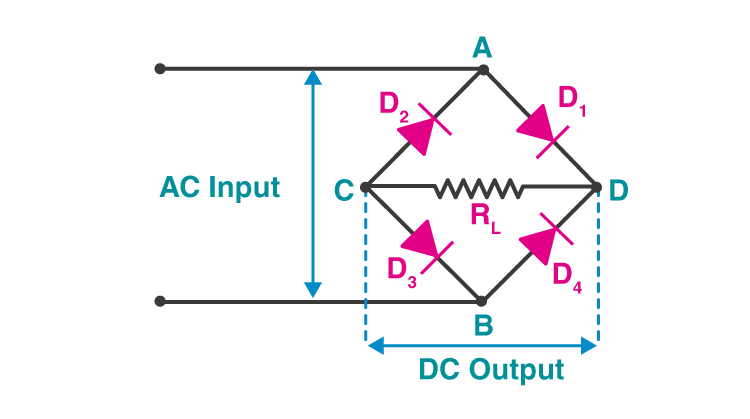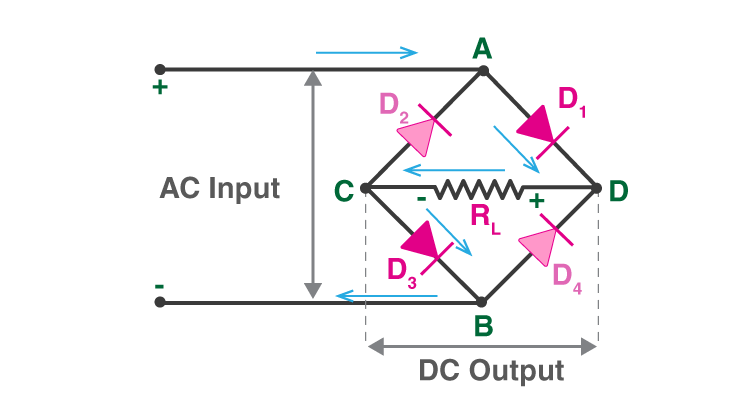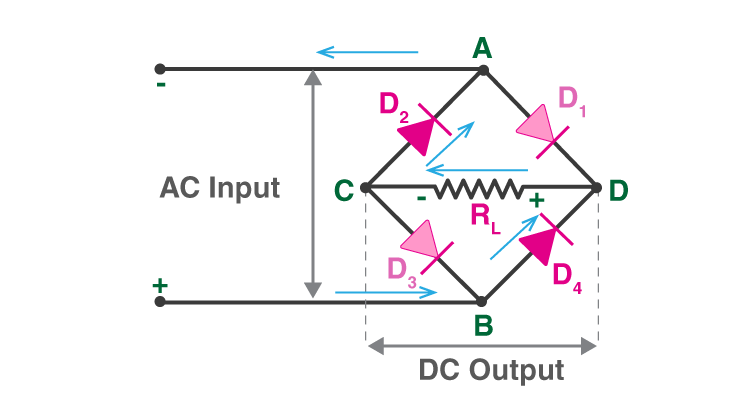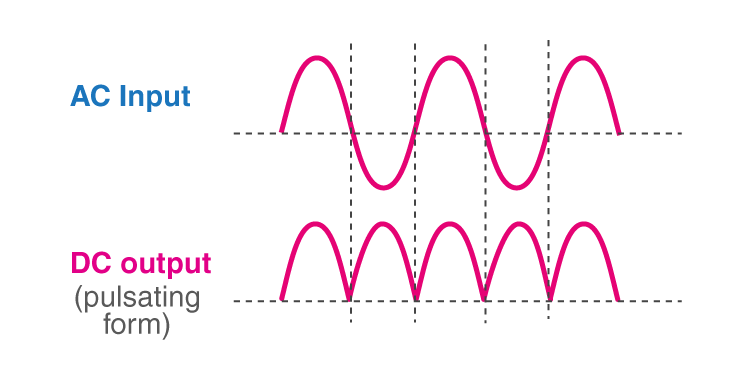# Bridge Rectifier

Many electronic circuits require a rectified DC power supply to power various electronic basic components from the available AC mains supply. Rectifiers are used to convert an AC power to a DC power. Among the rectifiers, the bridge rectifier is the most efficient rectifier circuit.

We can define bridge rectifiers as a type of full-wave rectifier that uses four or more diodes in a bridge circuit configuration to efficiently convert alternating (AC) current to a direct (DC) current. In the next few sections, let us learn more about its construction, working, and more.

## Construction

The construction of a bridge rectifier is shown in the figure below. The bridge rectifier circuit is made of four diodes D1, D2, D3, D4, and a load resistor RL. The four diodes are connected in a closed-loop configuration to efficiently convert the alternating current (AC) into Direct Current (DC). The main advantage of this configuration is the absence of the expensive centre-tapped transformer. Therefore, the size and cost are reduced.The input signal is applied across terminals A and B, and the output DC signal is obtained across the load resistor RL connected between terminals C and D. The four diodes are arranged in such a way that only two diodes conduct electricity during each half cycle. D1 and D3 are pairs that conduct electric current during the positive half cycle/. Likewise, diodes D2 and D4 conduct electric current during a negative half cycle.

## Working

When an AC signal is applied across the bridge rectifier, terminal A becomes positive during the positive half cycle while terminal B becomes negative. This results in diodes D1 and D3 becoming forward biased while D2 and D4 becoming reverse biased.

The current flow during the positive half-cycle is shown in the figure below:During the negative half-cycle, terminal B becomes positive while terminal A becomes negative. This causes diodes D2 and D4 to become forward biased and diode D1 and D3 to be reverse biased.

The current flow during the negative half cycle is shown in the figure below:From the figures given above, we notice that the current flow across load resistor RL is the same during the positive and negative half-cycles. The output DC signal polarity may be either completely positive or negative. In our case, it is completely positive. If the diodes’ direction is reversed, we get a complete negative DC voltage.

Thus, a bridge rectifier allows electric current during both positive and negative half cycles of the input AC signal.

The output waveforms of the bridge rectifier are shown in the below figure.## Characteristics of Bridge Rectifier

### Ripple Factor

The smoothness of the output DC signal is measured by a factor known as the ripple factor. The output DC signal with fewer ripples is considered a smooth DC signal while the output with high ripples is considered a high pulsating DC signal.

Mathematically, the ripple factor is defined as the ratio of ripple voltage to pure DC voltage.

The ripple factor for a bridge rectifier is given by

$$\begin{array}{l}\gamma =\sqrt{(\frac{{V_{rms}}^2}{V_{DC}})-1}\end{array}$$

For bridge rectifiers, the ripple factor is 0.48.

### Peak Inverse Voltage

The maximum voltage that a diode can withstand in the reverse bias condition is known as a peak inverse voltage. During the positive half cycle, the diodes D1 and D3 are in the conducting state while D2 and D4 are in the non-conducting state. Similarly, during the negative half cycle, diodes D2 and D4 are in the conducting state, and diodes D1 and D3 are in the non-conducting state.

### Efficiency

The rectifier efficiency determines how efficiently the rectifier converts Alternating Current (AC) into Direct Current (DC). Rectifier efficiency is defined as the ratio of the DC output power to the AC input power. The maximum efficiency of a bridge rectifier is 81.2%.

$$\begin{array}{l}\eta =\frac{DC\,Output\,Power}{AC\,Output\,Power}\end{array}$$

• The efficiency of the bridge rectifier is higher than the efficiency of a half-wave rectifier. However, the rectifier efficiency of the bridge rectifier and the centre-tapped full-wave rectifier is the same.
• The DC output signal of the bridge rectifier is smoother than the output DC signal of a half-wave rectifier.
• In a half-wave rectifier, only half of the input AC signal is used, and the other half is blocked. Half of the input signal is wasted in a half-wave rectifier. However, in a bridge rectifier, the electric current is allowed during both positive and negative half cycles of the input AC signal. Hence, the output DC signal is almost equal to the input AC signal.

• The circuit of a bridge rectifier is complex when compared to a half-wave rectifier and centre-tapped full-wave rectifier. Bridge rectifiers use 4 diodes while half-wave rectifiers and centre-tapped full wave rectifiers use only two diodes.
• When more diodes are used more power loss occurs. In a centre-tapped full-wave rectifier, only one diode conducts during each half cycle. But in a bridge rectifier, two diodes connected in series conduct during each half cycle. Hence, the voltage drop is higher in a bridge rectifier.

## Frequently Asked Questions – FAQs

YES.

### Define bridge rectifiers.

The bridge rectifier is a type of full-wave rectifier that uses four or more diodes in a bridge circuit configuration to convert alternating (AC) current to a direct (DC) current.

### What is a rectifier?

A rectifier is an electronic device that converts an alternating current into a direct current by using one or more P-N junction diodes.

TRUE.

### What is the maximum efficiency of a bridge rectifier?

The maximum efficiency of a bridge rectifier is 81.2%.

Stay Tuned to BYJU’S and FALL IN LOVE WITH LEARNING!

Test your knowledge on Bridge Rectifier